# Divide And Conquer Notes

Reference:

Note: We sometimes call it CDQ algorithm. CDQ is a name of a Chinese scholar.

Introduction to Algorithms describes Divide And Conquer as follows:

• Divide the problem into a number of independent subproblems that are smaller instances of the same problem.
• Conquer the subproblems by solving them recursively. If the subproblem sizes are small enough, however, just solve the subproblems in a straightforward manner.
• Combine the solutions to the subproblems into the solution for the original problem.

## Recurrence Relation

We can use recurrence relation to depict time complexity of divide and conquer:

Consider size of the original problem is $n$:

• The problem is divided into $a$ subproblems.
• Each subproblem size is $\frac{1}{b}$ of the original problem, so the size is $\frac{n}{b}$.
• Each subproblem takes $T(\frac{n}{b})$ time.
• Dividing takes $D(n)$.
• Merging or combining takes $C(n)$.

So, we have the recurrence relation:

$$T(n) = aT(\frac{n}{b}) + D(n) + C(n)$$

For example, as for mergesort:

• $a = b = 2$, $D(n) = \Theta(1)$, $C(n) = \Theta(n)$.
• $T(n) = \Theta(n\log{n})$

How do we know the total time complexity? The answer is to solve the recurrence.

## Three Methods

There are three methods: substitution method, recursion tree, master method

The substitution method is super powerful and it could be used to solve all recurrence relations, but it is kind of an overhead. As for some particular problems or formulas, we can use master method.

### Substitution Method

Two steps:

• Predict: Guess the form of the solution.
• Prove: Use mathematical induction to find the constants and show that the solution works.

### Recursion Tree

A recursion tree is useful for visualizing what happens when a recurrence is iterated. It diagrams the tree of recursive calls and the amount of work done at each call.

Mergesort:

Based on the recurrence $T(n) = 2T(\frac{n}{2}) + O(n)$, we have the recursion tree of mergesort as follows: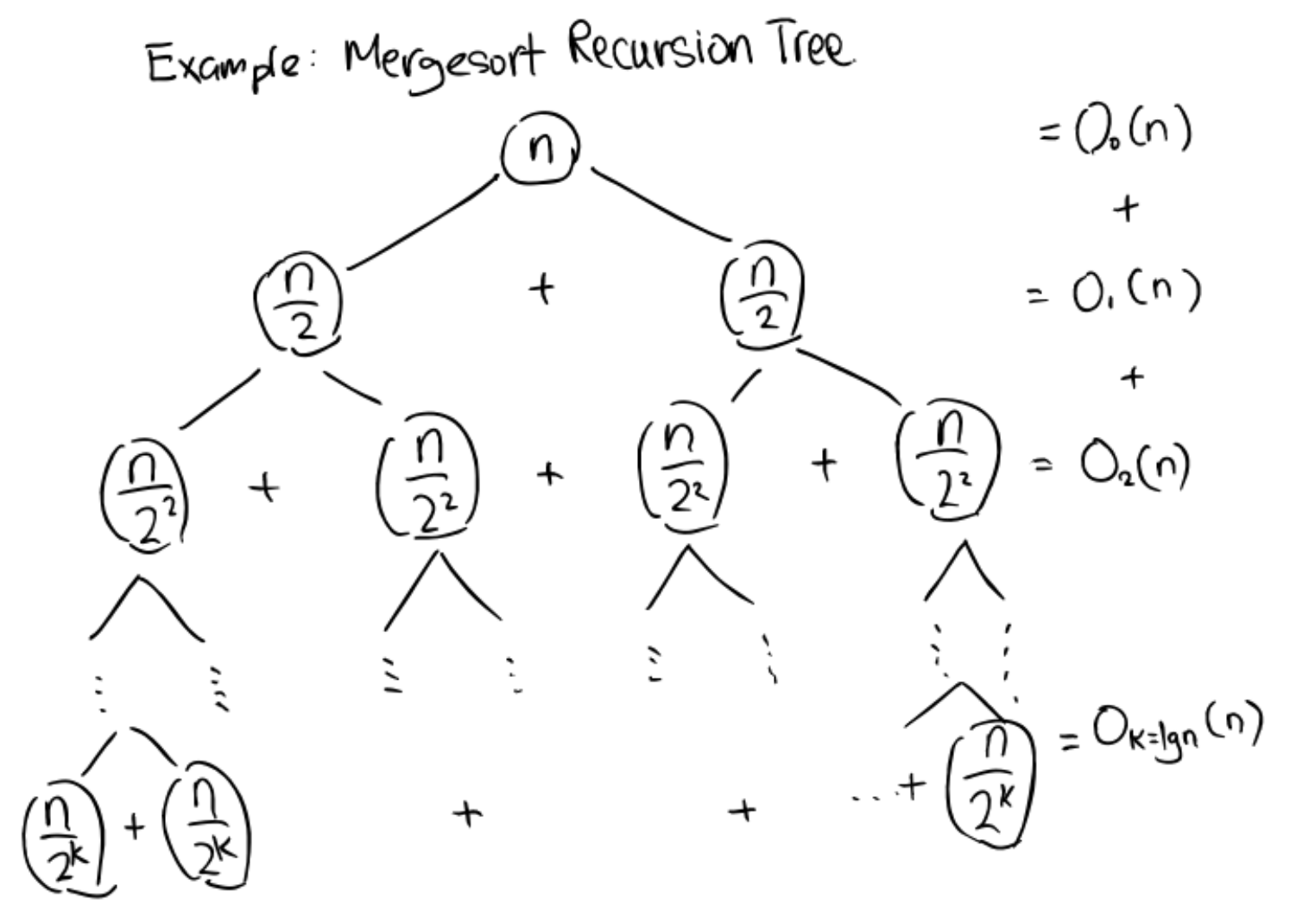According to the tree, we sum up $k$ of $\log{n}$ and have $O(n\log{n})$.

Squared Combination Time:

Consider the following recurrence: $T(n) = 2T(\frac{n}{2}) + n^2$. The tree is as follows: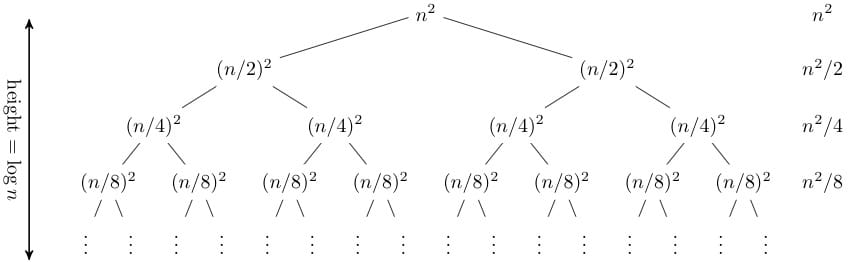In the second row, $(\frac{n}{2})^2 + (\frac{n}{2})^2 = \frac{n^2}{2}$.

\begin{aligned} T(n) &= O(n^2 \times (1 + \frac{1}{2} + \frac{1}{4} + \frac{1}{8} + \ldots + \frac{1}{2^{lg{n}}}))\ &= O(n^2 \times (1 + 1))\ &= O(n^2)\ \end{aligned}

The sum is $O(n^2)$. In this case, the depth of the tree does not really matter; the amount of work at each level is decreasing so quickly that the total is only a constant factor more than the root.

Note: Recursion trees can be useful for gaining intuition about a closed form, but they are not a proof. Sometimes it is easy to get the wrong answer.

A good way of establishing a closed form is to make an educated guess and then prove by induction. Therefore, recurrence trees can be a good method of guessing.

Consider another example: $T(n) = T(\frac{n}{3}) + T(\frac{2}{3}n)$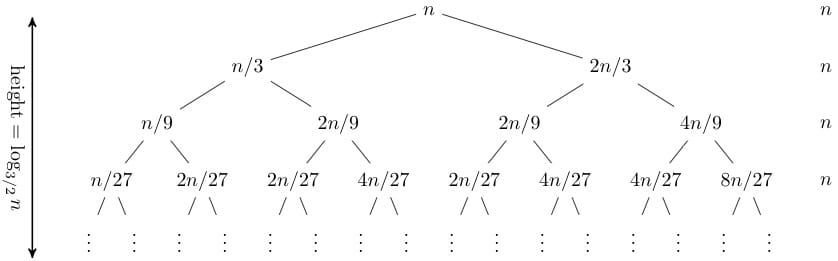Note that the tree here is not balanced. The longest path is the rightmost one, and its length is $\log_{3/2}{n}$, so our guess for the closed form of this recurrence is $O(n\log{n})$.

Fibonacci Series:

As for Fibonacci Series, the recurrence is $T(n) = T(n - 1) + T(n - 2) + O(1)$.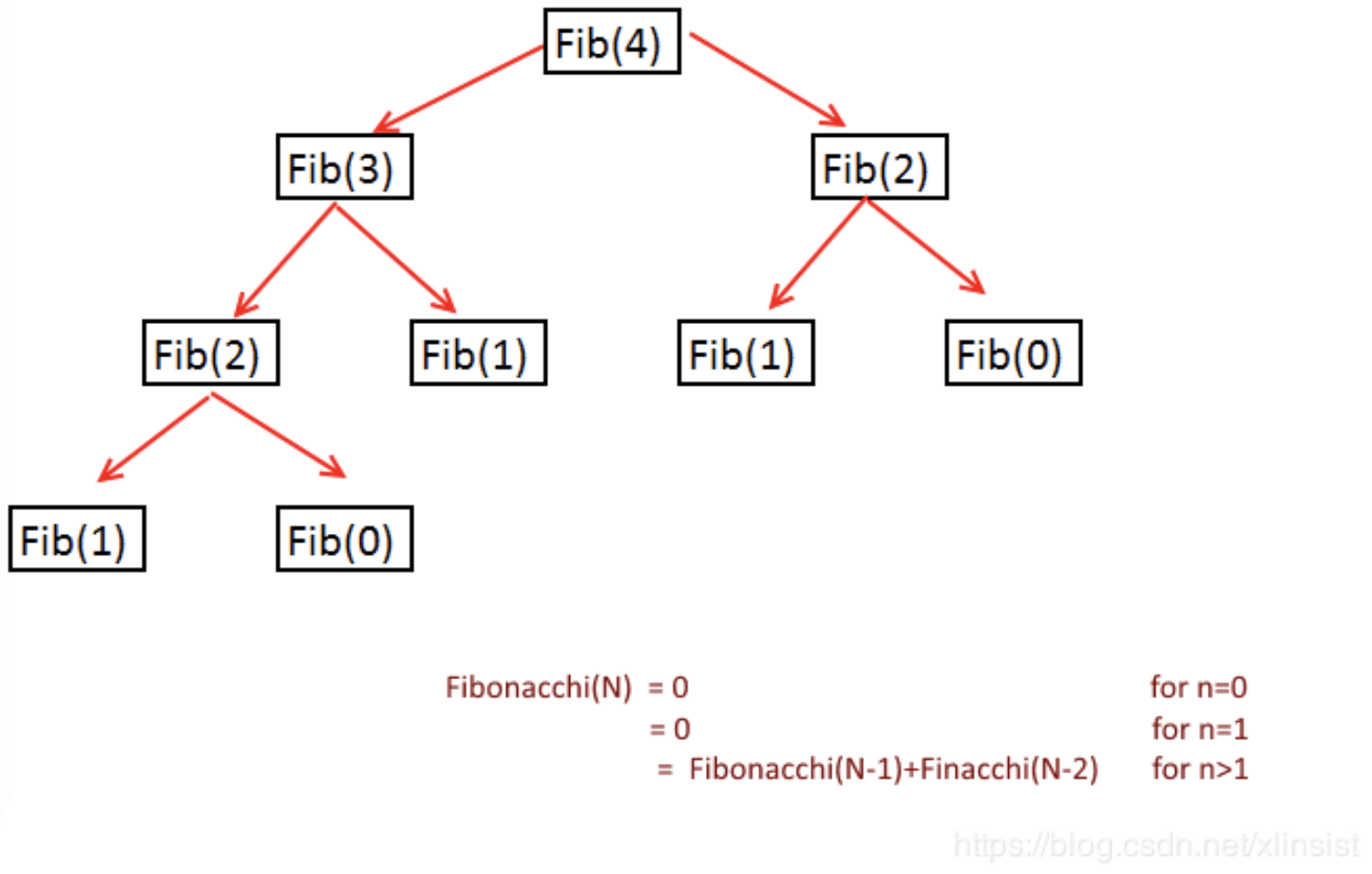According to the tree, the longest path is the leftmost one, so the depth is $O(n)$. The runtime is as follows:

\begin{aligned} T(n) &= O(1 + 2 + 4 + 8 + \ldots + 2^n)\ &= O(2 \times 2^n + 1)\ &= O(2^n)\ \end{aligned}

### The Master Method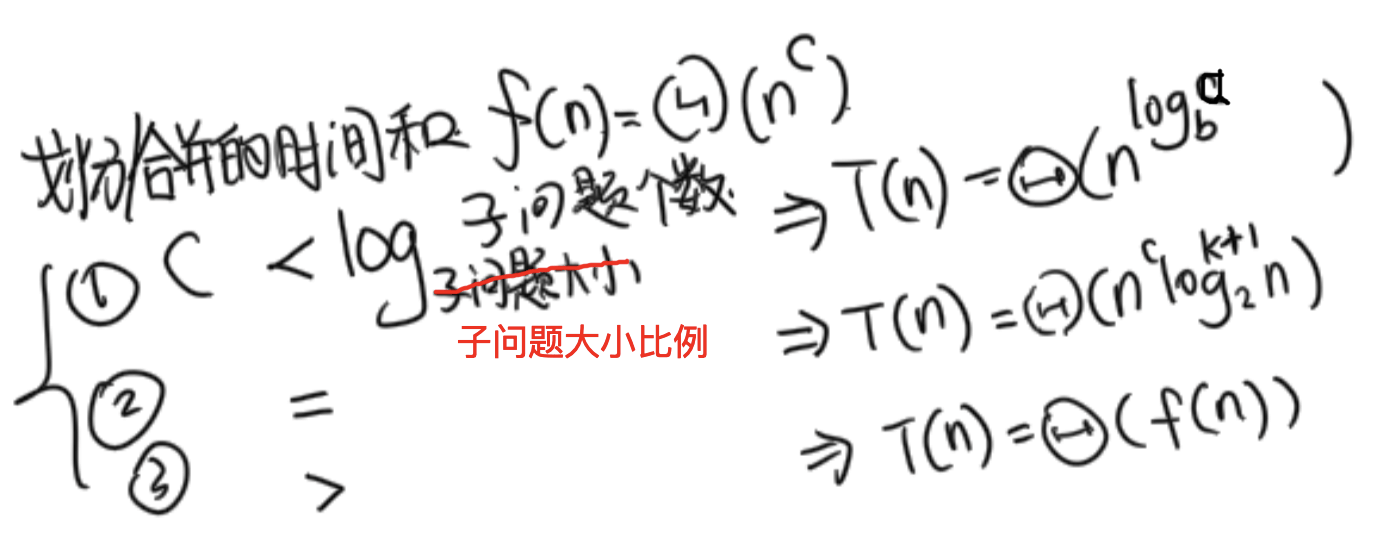Note: 上面 log 的底标错了，$n/b$ 才是子问题的大小，$b$ 只是一个比例。

The master theorem concerns recurrence relations of the form:

$$T(n) = aT(\frac{n}{b}) + f(n),\ \ \text{where}\ a \geq 1, b > 1$$

We can just simply apply the theorem to solve recurrences. There are three cases and we need to figure out in which case the recurrence is to apply the following formula.

Recall:

• $a$: #subproblems
• $n/b$: Size of the subproblem
• $f(n)$: Division and combination time

Case 1: $c < \log_b{a}$

• If:
• $f(n) = \Theta(n^c)$, where $c < \log_b{a}$ (using Big-O notation)
• Then:
• $T(n) = \Theta(n^{\log_b{a}})$

Case 2: $c = \log_b{a}$

• If: (for some constant $k \geq 0$)
• $f(n) = \Theta(n^c \log^k{n})$, where $c = \log_b{a}$
• Then:
• $T(n) = \Theta(n^c \log^{k + 1}{n})$

Case 3: $c > \log_b{a}$

• If:
• $f(n) = \Theta(n^c)$, where $c > \log_b{a}$
• Then:
• $T(n) = \Theta(f(n))$

Therefore, we just need to figure the relation between $a$, $b$, and $c$, then we can compute the time complexity for a specific case.

Note: If the following cases occur, we cannot apply the master theorem.

• $T(n)$ is not monotone, e.g. $T(n) = \sin(n)$
• $f(n)$ is not a polynomial, e.g. $T(n) = 2T(\frac{n}{2}) + 2^n$
• $b$ cannot be expressed as a constant, e.g. $T(n) = T(\sqrt{n})$

Examples: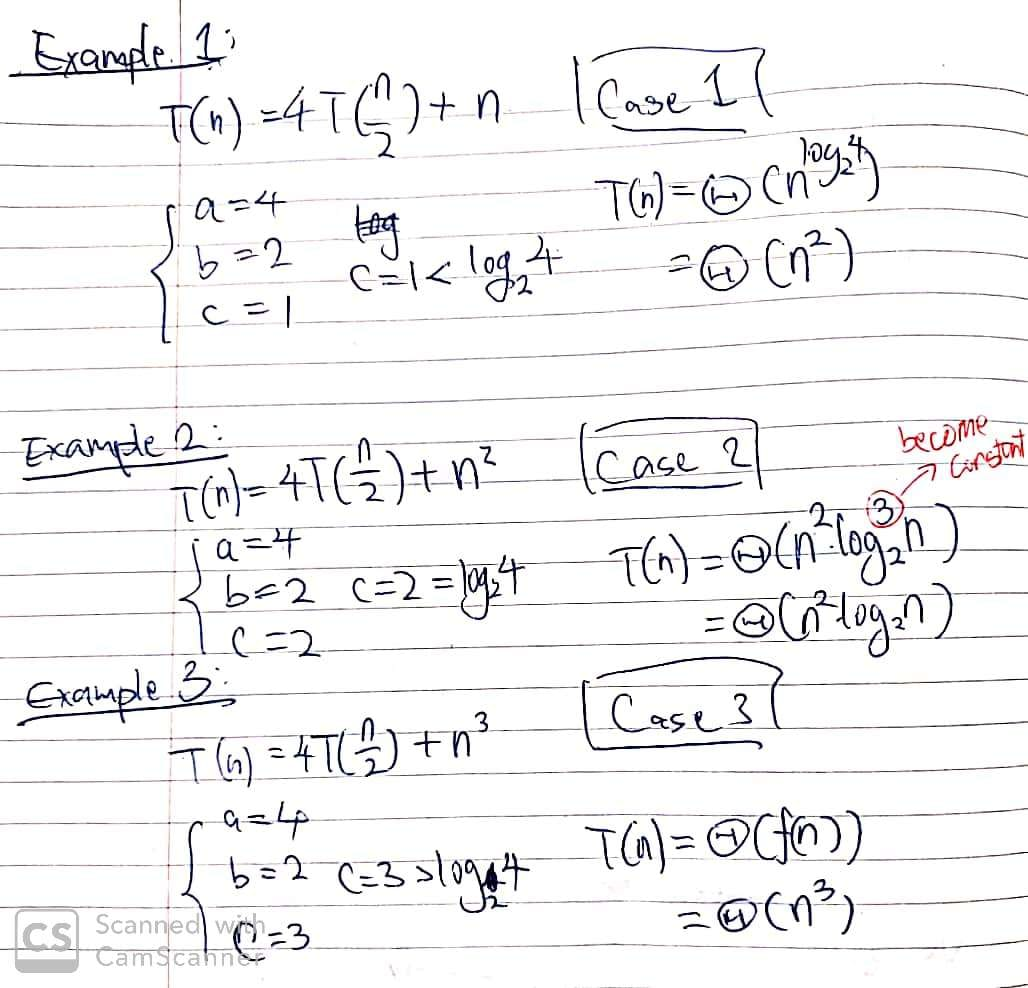CommentJunhao Wang
a software engineering cat